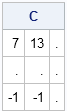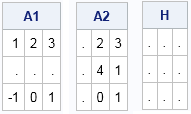Sometimes I get contacted by SAS/IML programmers who discover that the SAS/IML language does not provide built-in support for multiplication of matrices that have missing values. (SAS/IML does support elementwise operations with missing values.) I usually respond by asking what they are trying to accomplish, because mathematically matrix multiplication with missing values is not a well-defined operation. I get various answers. Some people want to exclude the missing values. Others want them propagated. Still others want them imputed.

This article discusses possible ways to multiply matrices when the matrices contain missing values. It defines a SAS/IML module that propagates missing values. The module can be used to score a linear model data that include missing values.

### Linear algebra with missing values? Does it make sense?

SAS software supports scalar operations on missing values. Multiplication that involves a missing value results in a missing value. Additive operations exclude (skip) the missing value. Many SAS procedures, including PROC IML, handle missing data in statistical calculations.

Linear algebra is another story. A vector space is defined over an algebraic field, which is a set of numbers that support addition, subtraction, multiplication, and division. Every element of the field must have an additive inverse, and every nonzero element must have a multiplicative inverse (reciprocal). A missing value does not have either. There is no number that can be added to a missing value to get zero. Similarly, there is no number such that the product of the number and a missing value is 1.

Consequently, you can't include missing values in matrices and expect to preserve the usual laws of linear algebra. However, you can define new operations on numerical arrays that are reminiscent of matrix multiplication. In the rest of this article, A and B are matrices where the number of columns of A equals the number of rows of B. Read on to discover ways to compute a "matrix product" C = A*B when either matrix has a missing value.

### Excluding rows with missing values

By far the most common way to handle missing values in a statistical analysis is to exclude them. See my previous blog post about how to perform listwise deletion of missing values in the DATA step and in SAS/IML.

### Propagating missing values

In some software packages (including MATLAB and R), missing values are propagated by matrix multiplication. If the matrix A has a missing value anywhere in the ith row, the product A*B contains missing values everywhere in the ith row. Similarly, if B has a missing value anywhere in the jth column, the entire jth column of the product A*B contains missing values.

It is easy to define a SAS/IML module that implements this multiplication scheme: Use ordinary matrix multiplication on rows and columns that are free of missing values and put missing values everywhere else. The following function implements this multiplication method:

```proc iml; /* matrix "multiplication" where missing values are propagated */ start MVMult(A, B); C = j(nrow(A), ncol(B), .); rows = loc(countmiss(A, "ROW")=0); cols = loc(countmiss(B, "COL")=0); if ncol(rows)>0 & ncol(cols)>0 then C[rows, cols] = A[rows,] * B[,cols]; return(C); finish;   A = {1 2 3, . 4 1, -1 0 1}; B = {1 2 -1, 3 4 0, 0 1 .}; C = MVMult(A,B); print C;```The product matrix contains a missing value for every element of the second row because the A matrix has a missing value in the second row. The product matrix contains a missing value for every element of the third column because the B matrix has a missing value in the third column.

This a reasonable way to handle missing values when you are trying to score data according to a linear model. In that case, the matrix on the left side is an n x p data matrix (X). Each column of the right-hand matrix (B) contains p coefficients of a linear model. Often the right-hand matrix is a column vector. The matrix product P=X*B evaluates (scores) the linear model to obtain predicted values of the response. A missing value anywhere in the ith row of X indicates that you cannot predict a value for that observation. When scoring linear models, the right-hand matrix does not usually contain missing values, although PROC SCORE permits missing value for coefficients and treats them as 0.

The previous paragraph shows why propagating missing values makes sense when scoring linear models. However, I prefer not to call it matrix multiplication. For example, in true matrix multiplication, a product that involves the identity matrix results in the original matrix. That does not hold true when missing values propagate. Furthermore, in a product of three matrices for which the middle matrix contains missing values, every element of the product is missing, which limits the usefulness of this technique.

```A1 = MVMult(A, I(3)); /* A*I ^= A */ A2 = MVMult(I(3), A); /* I*A ^= A */ H = MVMult(A2, I(3)); /* every element of I*A*I is missing */ print A1, A2, H;```### Skipping missing values

Some SAS customers have expressed interest in "skipping" missing values when they multiplying matrices. Recall that each element of a matrix product A*B is formed by multiplying elements of A and B and then summing those scalar products. It is reasonable to expect that multiplication of a missing value should result in a missing value, but that missing values are skipped in the summation, just as they are when using the SUM function.

You can define a SAS/IML module that implements this scheme, but this is not a good way to handle missing values. This scheme treats missing values as if they were zero. The following statements replace each missing value by 0, and then perform ordinary matrix multiplication. The product is the same as when you "skip" missing values during the summation:

```A0 = A; B0 = B; A0[ loc(A=.) ] = 0; /* replace missing values with 0 */ B0[ loc(B=.) ] = 0; C0 = A0*B0; print C0;```

Replacing missing values with 0 is a bad idea. Substituting the value 0 is likely to bias whatever analysis you are trying to perform. If you want to impute the missing values, you should use an imputation scheme that makes sense for the data and for the analysis.

### Conclusions

In conclusion, there are several ways to deal with multiplying matrices that contain missing values in SAS/IML software:

• Use only complete cases of the data by deleting any observation that contains a missing value.
• Propagate missing values by using the MVMult function in this blog post. This approach makes sense if you are evaluating a linear model on data that contain missing values.
• Impute the missing values. There are many ways to impute missing values in SAS, but imputing them with the value 0 is not usually a good choice. Consequently, I do not recommend that you skip missing values during matrix multiplication, which is equivalent to substitution by 0.
Share